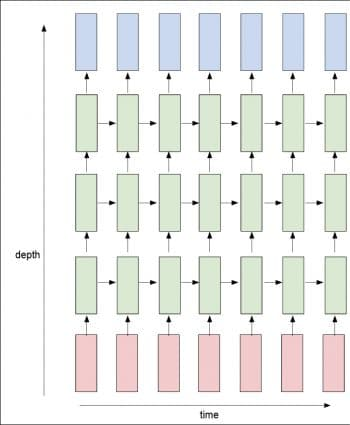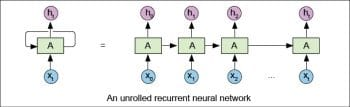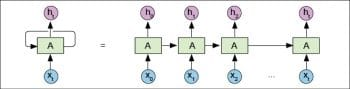# Using PyTorch for Natural Language Processing

0
2633This article is focused on the classes that PyTorch provides, which help with Natural Language Processing (NLP).

Natural language processing (NLP) is a subset of computer science, and is mainly about artificial intelligence (AI). It enables computers to understand and process human language. Technically, the main objective of NLP is to program computers for analysing and processing natural language data.

PyTorch is one of the most popular deep learning frameworks that is based on Python and is supported by Facebook. It is an open source machine learning library built on the Torch library, and used for applications such as computer vision and natural language processing. Developed by Facebook’s AI Research (FAIR) lab, it is free and open source software released under the modified BSD licence. Although the Python interface is more polished and the main focus of development, PyTorch also has a C++ interface. Some examples of PyTorch are Uber’s Pyro, HuggingFace’s Transformers, etc.

PyTorch provides two high-level characters:

• Tensor computing (like NumPy) with strong acceleration through graphics processing units (GPU)
• Deep neural networks built on a tape based automatic differentiation (autodiff) systemFigure 1: Recurrent neural network

How to use PyTorch for NLP

There are mainly six classes in PyTorch that can be used for NLP related jobs using recurrent layers:

• torch.nn.RNN
• torch.nn.LSTM
• torch.nn.GRU
• torch.nn.RNNCell
• torch.nn.LSTMCell
• torch.nn.GRUCell

Understanding these classes, their parameters, their inputs and their outputs is key to getting started with building your own neural networks for NLP in Pytorch.

Unrolled diagrams are used to provide simple-to-grasp explanations of the recurrent structure of such neural networks. Going from these pretty, unrolled diagrams and intuitive explanations to the Pytorch API can prove to be challenging.

Therefore, in this article, our objective is to bridge the gap by understanding the parameters, inputs, and outputs of the relevant classes in PyTorch in a clear and descriptive manner.

Pytorch basically has two levels of classes for building recurrent networks.

• Multi-layer classes  (nn.RNN, nn.GRU and nn.LSTM): Objects of these classes are capable of representing deep bi-directional recurrent neural networks [or, as the class names suggest, one or more of their evolved architectures  —  Gated Recurrent Unit (GRU) or Long Short Term Memory (LSTM) networks].
• Cell-level classes (nn.RNNCell, nn.GRUCell and nn.LSTMCell): Objects of these classes can represent only a single cell (again, a simple RNN, LSTM or GRU cell) that can handle one time step of the input data. Remember, these cells don’t have cuDNN optimisation and thus don’t have any fused operations, etc.

All the classes in the same level share the same API. Hence, understanding the parameters, inputs, and outputs of any one of the classes in both the above levels is sufficient. To understand them more easily, we will use the simplest classes  —  torch.nn.RNN and torch.nn.RNNCell.Figure 2: torch.nn.RNN

torch.nn.RNN

Let us look at Figure 2 to understand the API .

Parameters

This is the number of expected attributes in the input x. This represents the dimensions of vector x[i] (i.e., any of the vectors from x to x[t] in Figure 2).  Observe that it is simple to confuse this with the sequence length, which is the total number of cells that we get after unrolling the RNN, as seen above.

hidden_size:  This is the number of attributes in the hidden state h. This represents the dimension of vector h[i] (i.e., any of the vectors from h to h[t] in Figure 2). Jointly, input_size and hidden_size are necessary and sufficient to decide the shape of the weight matrices of the network.

num_layers: This is the number of recurrent layers; e.g., setting num_layers=2 would signify stacking two RNNs together to build a stacked RNN, with the second RNN taking in outputs of the first RNN and determining the final results.

The default value is set to 1 for the num_layers component. It comes under the num_layers parameter.

This parameter is used to build deep recurrent neural networks (RNNs) like in Figure 3.

In the figure, the pink cells represent the inputs, the light green blocks represent the RNN cells, and the light blue blocks represent the output. So, from the figure, we set the num_layer parameter as 3.

In the deep learning group, some people discovered that removing/using bias does not affect the model’s performance. Hence, this Boolean parameter.

• batch_first: If ‘True’, then the input and output tensors are provided as (batch, seq, feature). Default: False.
• dropout: If non-zero, this introduces a dropout layer on the outputs of each RNN layer except the last layer. Default: 0.
• bidirectional: If ‘True’, this becomes a bi-directional RNN. Default: False.

Note: To make RNN bi-directional, simply set the bi-directional parameter to ‘True’.

To make an RNN in PyTorch, we need to pass two mandatory parameters to the class es  input_size and hidden_size(h_0).

Inputs

input :  This is a tensor of shape (seq_len, batch, input_size). In order to work with variable length inputs, we pack the shorter input sequences.

h_0 : This is a tensor of shape (num_layers * num_directions, batch, hidden_size). num_directions is 2 for bi-directional RNNs and 1 otherwise.Figure 3: Deep RNNs

Outputs

In a similar manner, the object returns two outputs to us — output and h_n.

• output :  This is a tensor of shape (seq_len, batch, num_directions * hidden_size). It contains the output attribute (h_k) from the last layer of the RNN, for each k.
• h_n : This is a tensor of size (num_layers * num_directions, batch, hidden_size). It contains the hidden state for k = seq_len.

As stated earlier, both torch.nn.GRU and torch.nn.LSTM have the same API, i.e., they accept the same set of parameters and accept inputs in the same format, even returning them in the same format too.

torch.nn.RNNCell

After all, this represents only a single cell of the RNN, so it accepts only four parameters — all of which have the same meaning as they did in torch.nn.RNN.

Parameters

• input_size :  The number of expected features in the input x.
• hidden_size :  The number of features in the hidden state h.
• bias:  If ‘False’, then the layer does not use bias weights b_ih and b_hh. Default: True.
• non-linearity: This is the non-linearity to use. It can be either tanh or relu. Default: tanh.

Again, since this is just a single cell of an RNN, the input and output dimensions are much simpler .

Inputs

• input : This is a tensor of shape (batch, input_size) that contains the input features.
• hidden : This is a tensor of shape (batch, hidden_size) that contains the initial hidden states for each of the elements in the batch.

Output

h: This is a tensor of shape (batch, hidden_size) and it gives us the hidden state for the next time step.